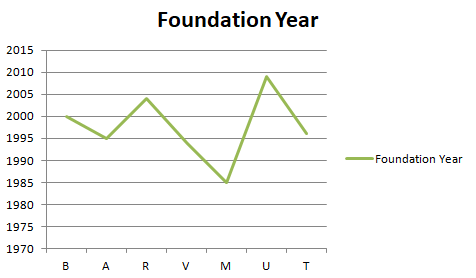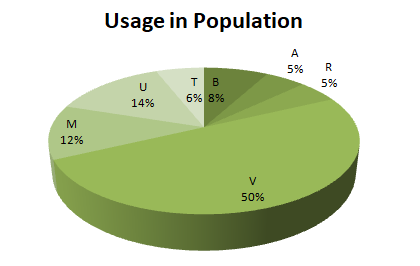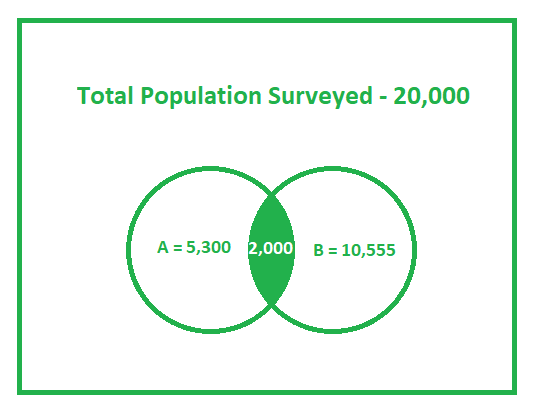GeeksforGeeks App
Open AppBrowser
Continue

# GRE Data Analysis | Data Interpretation Examples

Data Interpretation simply means to understand given data and transforming the same data into the desired property. Data given can be in any form from below given data representations

• Table
• Circular Graph
• Venn Diagram
• Bar Graph
• Scattered Plots
• Line Graphs

Approximately three Data Interpretation questions per section are asked in GRE and it can be of any type either MCQ or Numeric Entry.

### Data represented in Table Form:

The table shows a comparison of two plans(call charges for 1 minute and call charges for 2 minutes) provided by telecom companies. Please answer questions as per the table.

Telecom CompaniesCall Charge per minuteCall Charge per 2 minutes
B0.941
A0.981
R0.980.98
V0.950.98
M22.2
U1.11.3
T1.82.0

Ques-1:
Which company provides highest percentage increase in call charges from one minute plan to two minutes plan ?

Explanation:
For B, the Percentage increase = 0.06/0.94 = 6/946% (slightly greater than 6%)
For A, the Percentage increase = 0.02/0.98 = 2/982% (Slightly greater than 2%)
For R, there is no increase in the rates.
For V, the Percentage Increase = 0.03/0.95 = 3/953% (Slightly greater than 3%)
For M, the Percentage Increase = 0.2/2 = 1/10 = 10%
For U, the Percentage Increase = 0.2/1.1 = 2/1120% (Slightly less than 20%)
For T, the Percentage Increase = 0.2/1.8 = 2/18 = 11%

So the answer is U with 20% increase.

Ques-2:
How many companies increased charges in second plan greater than 10% ?

Explanation:
For B, the Percentage increase = 0.06/0.94 = 6/946% (slightly greater than 6%)
For A, the Percentage increase = 0.02/0.98 = 2/982% (Slightly greater than 2%)
For R, there is no increase in the rates.
For V, the Percentage Increase = 0.03/0.95 = 3/953% (Slightly greater than 3%)
For M, the Percentage Increase = 0.2/2 = 1/10 = 10%
For U, the Percentage Increase = 0.2/1.1 = 2/1120% (Slightly less than 20%)
For T, the Percentage Increase = 0.2/1.8 = 2/18 = 11%

Ques-3:
Based on the information in the table, which of the following three statements are true?

Explanation:
Statement 1: Percentage increase in charges of the second plan of M and T are the same.
Statement 2: M provides costly services amongst them all.
Statement 3: All companies provide less than 20% difference in both the plans.

• Statement-1: FALSE
Bases are different in both cases. Quantity with the higher base provides more percentage increase. In our case, it is M. Thus the statement is False.
• Statement-2: TRUE
Percentage increase, as well as the base of M, are highest. Thus it provides costly service amongst them all.
• Statement-3: TRUE
Increase in M rates are highest and also the percentage increase is highest which is slightly less than 20. Thus the statement is True.

### Data represented in Circular Graph and Line Graph:Above is mentioned the graph of Foundation years of different Telecom companies in a particular area and their usage in particular section. The total population of the section is 5, 00, 000.

Ques-1:
How many people use oldest telecom services?

Explanation:
The oldest telecom company is M having been founded in 1985.
From the pie chart, we can say that 12% of the population use telecom services provided by M.
Thus the answer is = 5, 00, 000 * (12/100) = 60, 000

Ques-2:
The companies founded after 2000 cover how much percentage of population ?

Explanation:
There are only two companies founded after 2000, which are R and U. So, the percentage of the population covered by these companies are (14 + 5) = 19%

### Data represented in Venn Diagram:

In a survey of 20, 000 people, 5, 300 have used telecom services provided by A; 10, 555 used telecom services provided by B and 2, 000 people have used services provided by both the providers.Ques-1:
How many people are using telecom services provided by A but not B ?

Explanation:
People using services provided by A – People using services provided by both

```= 5300 – 2000
= 3300 ```

Ques-2:
How many people are using services provided by at least one service provider ?

Explanation:
People using services provided by A + People using services provided by B – People using services provided by both

```= 5300 + 10555 – 2000
= 13855 ```

Ques-3:
How many people do not use services provided by either of the service providers ?

Explanation:
Total – People using at least one service,

```= 20000 – 13855
= 6145 ```
My Personal Notes arrow_drop_up